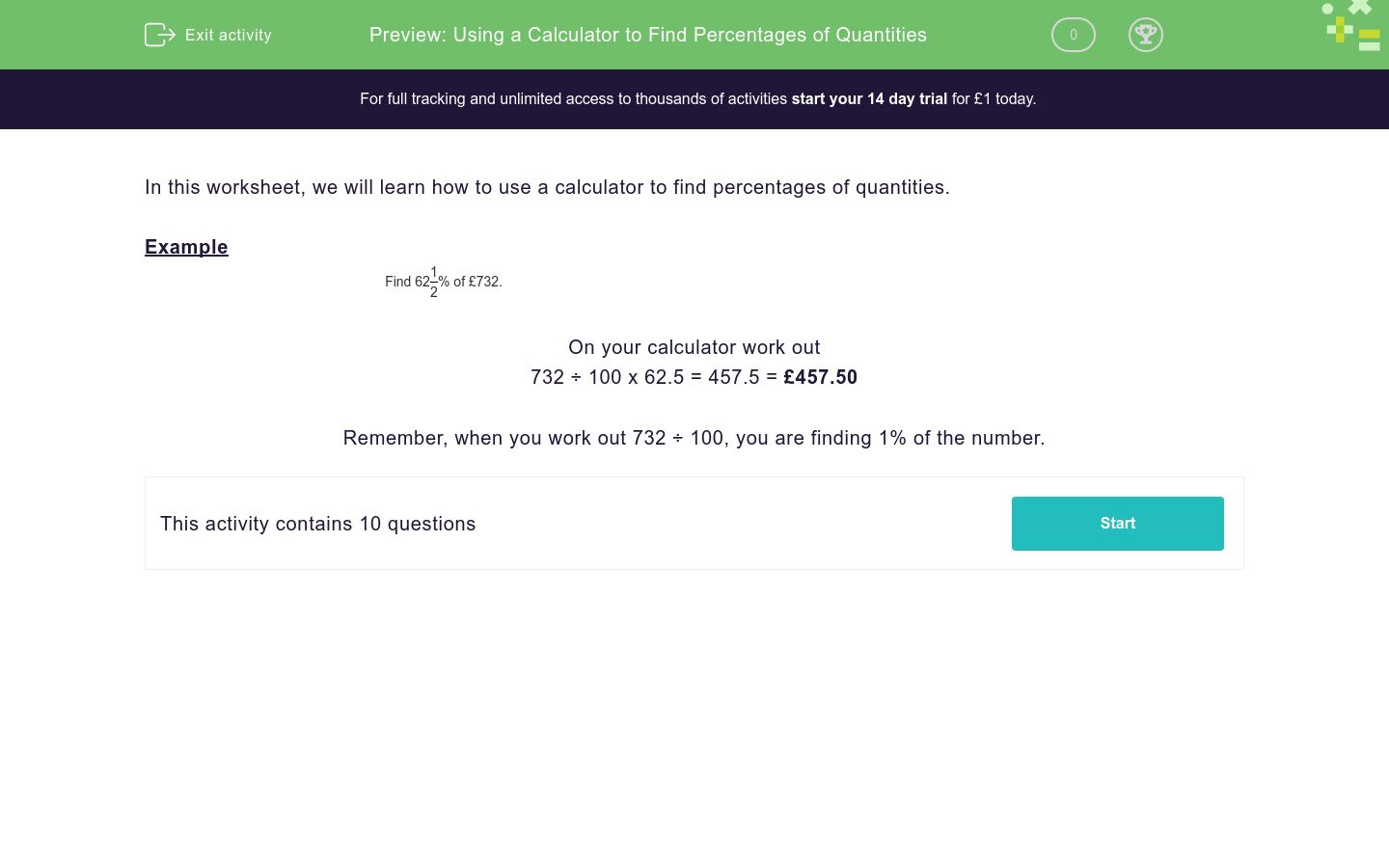# Using a Calculator to Find Percentages of Quantities

In this worksheet, students use a calculator to find percentages of quantities.Key stage:  KS 3

Curriculum topic:   Number

Curriculum subtopic:   Use Calculators/Technology for Accuracy

Difficulty level:### QUESTION 1 of 10

In this worksheet, we will learn how to use a calculator to find percentages of quantities.

Example

Find 62
 1 2
% of £732.

732 ÷ 100 x 62.5 = 457.5 = £457.50

Remember, when you work out 732 ÷ 100, you are finding 1% of the number.

Use a calculator to work out:

134
 1 2
% of 168 km
=  ________ km

Use a calculator to work out:

28
 1 2
% of 920 km
=  ________ km

Use a calculator to work out:

5
 1 2
% of 246 km
=  ________ km

Use a calculator to work out:

17
 1 2
% of 862 g
=  ________ g

Use a calculator to work out:

63
 1 2
% of 540 g
=  ________ g

Use a calculator to work out:

50
 1 2
% of £240
=  £ ________

Use a calculator to work out:

27
 1 2
% of £588
=  £ ________

Use a calculator to work out:

7
 1 2
% of £101
=  £ ________

Use a calculator to work out:

55
 1 2
% of £240
=  £ ________

Use a calculator to work out:

97
 1 2
% of £588
=  £ ________
• Question 1

Use a calculator to work out:

134
 1 2
% of 168 km
=  ________ km
225.96
• Question 2

Use a calculator to work out:

28
 1 2
% of 920 km
=  ________ km
262.2
• Question 3

Use a calculator to work out:

5
 1 2
% of 246 km
=  ________ km
13.53
• Question 4

Use a calculator to work out:

17
 1 2
% of 862 g
=  ________ g
150.85
• Question 5

Use a calculator to work out:

63
 1 2
% of 540 g
=  ________ g
342.9
• Question 6

Use a calculator to work out:

50
 1 2
% of £240
=  £ ________
121.20
• Question 7

Use a calculator to work out:

27
 1 2
% of £588
=  £ ________
161.70
• Question 8

Use a calculator to work out:

7
 1 2
% of £101
=  £ ________

7.58
• Question 9

Use a calculator to work out:

55
 1 2
% of £240
=  £ ________
133.20
• Question 10

Use a calculator to work out:

97
 1 2
% of £588
=  £ ________
573.30
---- OR ----

Sign up for a £1 trial so you can track and measure your child's progress on this activity.

### What is EdPlace?

We're your National Curriculum aligned online education content provider helping each child succeed in English, maths and science from year 1 to GCSE. With an EdPlace account you’ll be able to track and measure progress, helping each child achieve their best. We build confidence and attainment by personalising each child’s learning at a level that suits them.

Get started